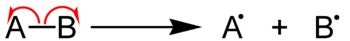# 18.2: Homolysis

$$\newcommand{\vecs}{\overset { \rightharpoonup} {\mathbf{#1}} }$$ $$\newcommand{\vecd}{\overset{-\!-\!\rightharpoonup}{\vphantom{a}\smash {#1}}}$$$$\newcommand{\id}{\mathrm{id}}$$ $$\newcommand{\Span}{\mathrm{span}}$$ $$\newcommand{\kernel}{\mathrm{null}\,}$$ $$\newcommand{\range}{\mathrm{range}\,}$$ $$\newcommand{\RealPart}{\mathrm{Re}}$$ $$\newcommand{\ImaginaryPart}{\mathrm{Im}}$$ $$\newcommand{\Argument}{\mathrm{Arg}}$$ $$\newcommand{\norm}{\| #1 \|}$$ $$\newcommand{\inner}{\langle #1, #2 \rangle}$$ $$\newcommand{\Span}{\mathrm{span}}$$ $$\newcommand{\id}{\mathrm{id}}$$ $$\newcommand{\Span}{\mathrm{span}}$$ $$\newcommand{\kernel}{\mathrm{null}\,}$$ $$\newcommand{\range}{\mathrm{range}\,}$$ $$\newcommand{\RealPart}{\mathrm{Re}}$$ $$\newcommand{\ImaginaryPart}{\mathrm{Im}}$$ $$\newcommand{\Argument}{\mathrm{Arg}}$$ $$\newcommand{\norm}{\| #1 \|}$$ $$\newcommand{\inner}{\langle #1, #2 \rangle}$$ $$\newcommand{\Span}{\mathrm{span}}$$$$\newcommand{\AA}{\unicode[.8,0]{x212B}}$$

In chemistry , homolysis (from Greek ὅμοιος, homoios, “equal,” and λύσις, lusis, “loosening”) or homolytic fission is chemical bond dissociation of a molecule by a process where each of the fragments retains one of the originally bonded electrons. During homolytic fission of a neutral molecule with an even number of electrons, two free radicals will be generated.  That is, the two electrons involved in the original bond are distributed between the two fragment species. The energy involved in this process is called bond dissociation energy . Bond cleavage is also possible by a process called heterolysis .Because the relatively high energy required to break bonds in this manner, homolysis only occurs under certain circumstances:

• Ultraviolet radiation (e.g. sunlight causing sunburns)
• Heat
• Certain intramolecular bonds, such as the O–O bond of a peroxide, are weak enough to spontaneously homolytically dissociate with a small amount of heat.
• High temperatures in the absence of oxygen (pyrolysis) can induce homolytic elimination of carbon compounds.

## References

1. IUPAC, Compendium of Chemical Terminology, 2nd ed. (the “Gold Book”) (1997). Online corrected version:  (2006–) “homolysis (homolytic)“.